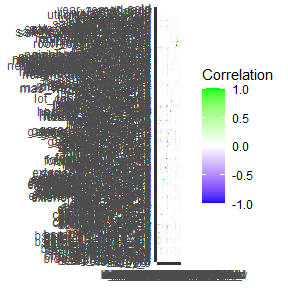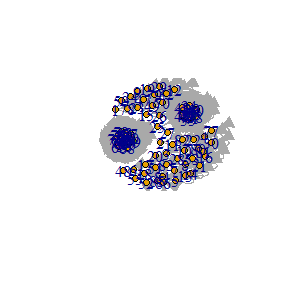# Introduction

In this walkthrough, we will go through the various use cases of TangledFeatures and how it outperforms traditional feature selection methods. We will also compare the raw accuracy of the package to other methods including standard black box approaches.

TangledFeatures relies on the dataset inputted to be correct. If there are categorical features please ensure that it is inputted as a factor. If done correctly, the package will identify the correct correlation based relationships between the variables.

In this example, we will be using the Boston housing prices data set. There has been some cleaning beforehand so that all the variables are in the correct orientation.

# Getting Started

TangledFeatures primarily relies on ggplot2 for visualization, data.table for data manipulation, correlation for various correlation methods and ranger for its Random Forest implementation. There are a few other packages used to run certain features.

library(ranger)
library(igraph)
#>
#> Attaching package: 'igraph'
#> The following objects are masked from 'package:stats':
#>
#>     decompose, spectrum
#> The following object is masked from 'package:base':
#>
#>     union
library(correlation)
library(data.table)
library(fastDummies)
library(ggplot2)

To read the data we can use the simple:

data <- TangledFeatures::Housing_Prices_dataset

This loads the base Boston housing prices data set. You can load this into the TangledFeatures function by:

# Correlation and treating the data

We are able to identify the variable class for each variable and assign it’s appropriate correlation relationship with other variables. Read more in the Correlation section.

If data_clean is set to ‘TRUE’ we follow a set of steps to clean the data including cleaning column names, dummy column creation and removal of NAs. Please read more in the ‘Data_input’ section.

Let’s set the function and call it’s various outputs

Results <- TangledFeatures::TangledFeatures(Data = TangledFeatures::Housing_Prices_dataset, Y_var = 'SalePrice', Focus_variables = list(), corr_cutoff = 0.7, RF_coverage = 0.95,  plot = TRUE, fast_calculation = FALSE, cor1 = 'pearson', cor2 = 'polychoric', cor3 = 'spearman')

The function returns visualizations and metrics about the correlation as well as other interesting metrics

Heatmap_plot <- Results$Correlation_heatmap plot(Heatmap_plot)For visualization purposes, we are only working with the top 20 variables. As we can see we can take the various relationships and visualize them on a single graph. TangledFeatures also returns the variables as well as the correlation metric used between them. Igraph_plot <- Results$Graph_plot
plot(Igraph_plot)As we can see from the graph, using graph theory algorithms we can trace the interrelationships between the variables. There are two distinct groups that we can see that have about the same effect on the sales price. We can take a single variable from each group as the group representative.

#here we are going to see the plot summary of this system